SELF 10 S.B. Karavashkin and O.N. Karavashkina5. Linear model of the electron’s excited motion under the external force affection Given the described mutual affection, the scheme of forces affecting the electron in the reference frame of the nucleus will take the appearance shown in Fig. 9.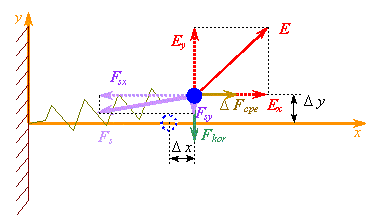Fig. 9. The forces affecting the orbital electron when it is excited by an external field
 Basing on the construction shown in Fig. 9, the modelling system of equations will take the following form: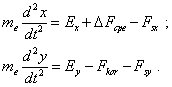(65)
 Substituting in (65) the values of involved forces from (55), (61), (63), (64), yield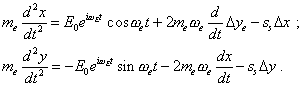(66)
 To find the solutions of (66), introduce two quite obvious substitutions. First, given in the left part there are present the derivatives of momentary coordinates of the electron, substitute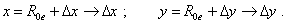(67)
 Second, let us write the expressions for the projections of the external force as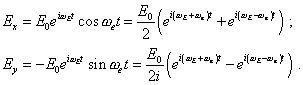(68)
 With (68) we see, the electron’s shift from the balance point is proportional to two time regularities - this means, we have to seek the solution as two summands: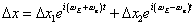(69)
 and the same in the axis y: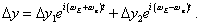(70)
 With it (66) bifurcates into two systems of equations: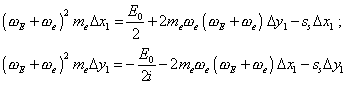(71)
 and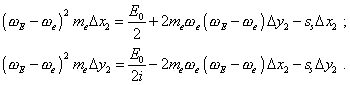(72)
 Both systems (71) and (72) have solutions that we can easily find. Solving (71), yield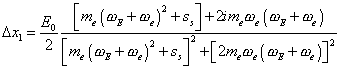(73)
 and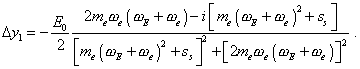(74)
 The same,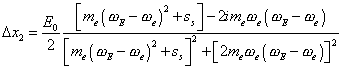(75)
 and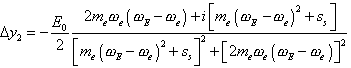(76)
 will be the solution of (72). Thus, general solution will be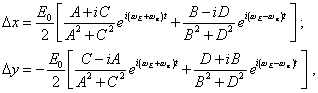(77)
 where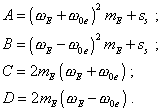Contents: / 1 / 2 / 3 / 4 / 5 / 6 / 7 / 8 / 9 / 10 / 11 / 12 / 13 / 14 / 15 /